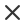FiltrTyp média
Typ licence
Orientace
Styl
Neobsahuje
Maths doodle hand drawn mathematics formulas on chalkboard for background banner book cover and etc education industry mathematical theory of school educationSeamless pattern on the theme of the subject of geometry formulas and charts of theorems a bright outline on a blue backgroundSketch of math class with working little people doodle cute miniature of teamwork and science symbols hand drawn cartoon vector illustration for school subject designMath education vector pattern with handwritten formulas tasks plots calculations and geometrical figures vector illustrationLine set maths in cartoon style design set featuring black and white math lesson illustrations utilizing sharp lines for clear and engaging educational visuals vector illustrationScientific formulas and calculations in physics and mathematics on whiteboard the lesson of algebra and geometry in school education and science engraved hand drawn in old sketch and vintage styleMath linear mathematics education circle background with geometrical plots formulas and calculations design concept vector illustrationSeamless pattern on the theme of the subject of geometry formulas and charts of theorems a bright outline on a dark backgroundMath formula mathematics calculus on school blackboard algebra and geometry science chalk pattern vector education concept scientific analysis number calculation complex knowledgeMath theory mathematics calculus on class chalkboard algebra and geometry science handwritten formulas vector education concept formula and theory on blackboard science study illustrationYoung woman sitting on the floor and solving math problem with black board female person dressed in casual clothes study at home vector flat color illustrationPhysical vector seamless pattern with scientific formulas research equations and figures handwritten with chalk on a grey board seamless designMathematic vector seamless pattern with different signs figures and formulas back to school seamless scientific backgroundMathematics textbook and formulas background vector open math book on green chalkboard backdrop with equations scientific signs and symbols school college or university education learn scienceDoodle math algebra and geometry school equation and graphs hand drawn physics science formulas vector image formulas education sketch for student homeworkMath education vector pattern with handwritten formulas tasks plots calculations and geometrical figures vector illustrationAn illustration of the word math written on pieces of colored paper pinned to a cork bulletin board vector eps 10 availableMath linear mathematics education circle background with geometrical plots formulas and calculations design concept vector illustrationPhysical formulas and phenomenon hand drawn illustration science board with math physics education at school vector illustrationBlue book with word mathematics icon isolated on green background math book education concept about back to school vector illustrationCreative vector illustration of math equation mathematical arithmetic physics formulas background art design screen blackboard template abstract concept graphic elementMathematical vector seamless pattern with angle protractors triangles drawing rulers formulas and calculations on a green backgroundAbstract seamless pattern with black handwritten text mathematical formulas and equations and geometrical figures vector repeating background in style from the diary of a scientistProgramming technical seamless pattern with programming code program flow diagrams formulas technical devices and schemes endless computer texture you can use any color of backgroundSchool seamless vector doodle pattern with different mathematical formulas and values good background for school printed supplies stationery other education stuff